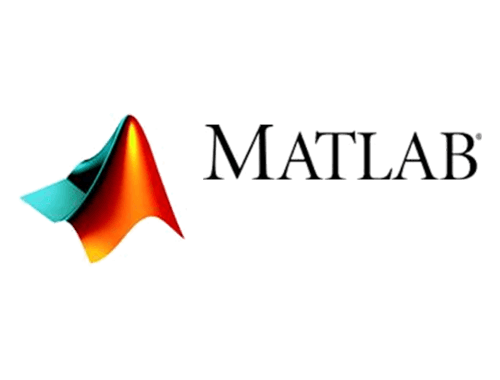# MATLAB

## Site License Information

• Name: MATLAB
• Eligible Users: TTU, Students, Faculty and Staff
• Operating System(s): PC, Mac
• Learn more at the official website

## How to Obtain and Other Information

MATLAB® is a high-level technical computing language and interactive environment for algorithm development, data visualization, data analysis, and numeric computation. Using the MATLAB product, you can solve technical computing problems faster than with traditional programming languages, such as C, C++, and Fortran.

You can use MATLAB in a wide range of applications, including signal and image processing, communications, control design, test and measurement, financial modeling and analysis, and computational biology. Add-on toolboxes (collections of special-purpose MATLAB functions) extend the MATLAB environment to solve particular classes of problems in these application areas.

MATLAB provides a number of features for documenting and sharing your work. You can integrate your MATLAB code with other languages and applications, and distribute your MATLAB algorithms and applications.

• High-level language for technical computing
• Development environment for managing code, files, and data
• Interactive tools for iterative exploration, design, and problem solving
• Mathematical functions for linear algebra, statistics, Fourier analysis, filtering, optimization, and numerical integration
• 2-D and 3-D graphics functions for visualizing data
• Tools for building custom graphical user interfaces
• Functions for integrating MATLAB based algorithms with external applications and languages, such as C, C++, Fortran, Java, COM, and Microsoft Excel

MATLAB is available from MathWorks through the eRaider website.

Note: Linux users will use the Windows activation key.

Detailed installation instructions